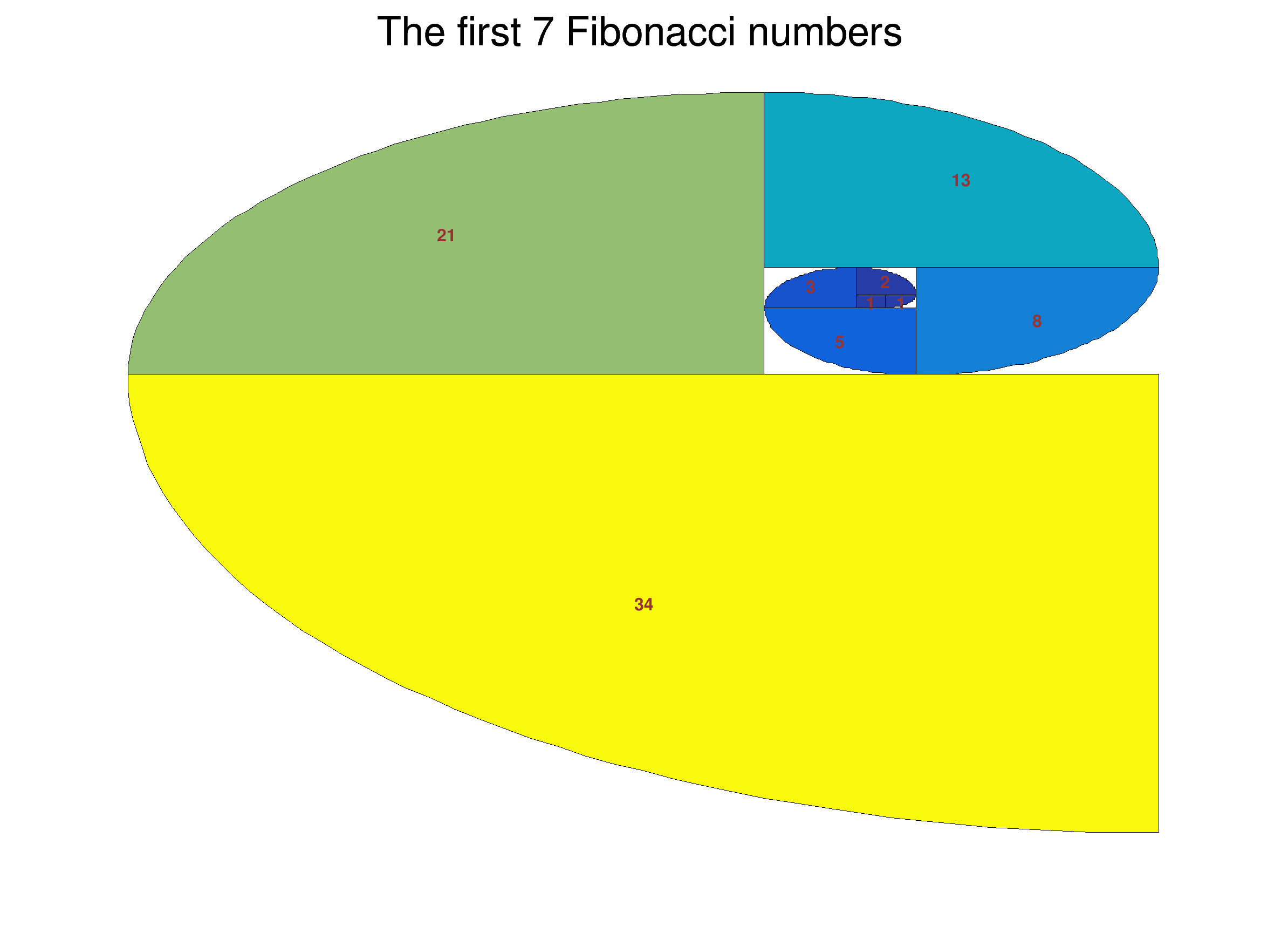ROOT   Reference GuideFibonacci.C File Reference

## Detailed Description

In mathematics, the Fibonacci sequence is a suite of integer in which every number is the sum of the two preceding one.

The first 10 Fibonacci numbers are:

1, 1, 2, 3, 5, 8, 13, 21, 34, 55, ...

This tutorial computes Fibonacci numbers and uses them to build a TH2Poly producing the "Fibonacci spiral" created by drawing circular arcs connecting the opposite corners of squares in the Fibonacci tiling.void Arc(int n, double a, double r, double *px, double *py);
void Fibonacci(int N=7) {
// N = number of Fibonacci numbers > 1
TCanvas *C = new TCanvas("C", "C", 800, 600);
C->SetFrameLineWidth(0);
TH2Poly *h2pf = new TH2Poly(); // TH2Poly containing Fibonacci bins.
h2pf->SetTitle(Form("The first %d Fibonacci numbers",N));
h2pf->SetMarkerColor(kRed-2);
h2pf->SetStats(0);
double f0 = 0.;
double f1 = 1.;
double ft;
for (int i=0; i<=N; i++) {
ft = f1;
f1 = f0 + f1;
f0 = ft;
}
h2pf->Draw("A COL L TEXT");
}
void Arc(int n, double a, double r, double *px, double *py) {
// Add points on a arc of circle from point 2 to n-2
double da = TMath::Pi()/(2*(n-2)); // Angle delta
for (int i = 2; i<=n-2; i++) {
a = a+da;
px[i] = r*TMath::Cos(a) + px;
py[i] = r*TMath::Sin(a) + py;
}
}
void AddFibonacciBin(TH2Poly *h2pf, double N) {
// Add to h2pf the bin corresponding to the Fibonacci number N
double X1 = 0.; //
double Y1 = 0.; // Current Fibonacci
double X2 = 1.; // square position.
double Y2 = 1.; //
static int MoveId = 0;
static double T = 1.; //Current Top limit of the bins
static double B = 0.; //Current Bottom limit of the bins
static double L = 0.; //Current Left limit of the bins
static double R = 1.; //Current Right limit of the bins
const int NP = 50; // Number of point to build the current bin
double px[NP]; // Bin's X positions
double py[NP]; // Bin's Y positions
double pi2 = TMath::Pi()/2;
switch (MoveId) {
case 1:
R = R+N;
X2 = R;
Y2 = T;
X1 = X2-N;
Y1 = Y2-N;
px = X1;
py = Y2;
px = X1;
py = Y1;
px[NP-1] = X2;
py[NP-1] = Y2;
Arc(NP,3*pi2,(double)N,px,py);
break;
case 2:
T = T+N;
X2 = R;
Y2 = T;
X1 = X2-N;
Y1 = Y2-N;
px = X1;
py = Y1;
px = X2;
py = Y1;
px[NP-1] = X1;
py[NP-1] = Y2;
Arc(NP,0.,(double)N,px,py);
break;
case 3:
L = L-N;
X1 = L;
Y1 = B;
X2 = X1+N;
Y2 = Y1+N;
px = X2;
py = Y1;
px = X2;
py = Y2;
px[NP-1] = X1;
py[NP-1] = Y1;
Arc(NP,pi2,(double)N,px,py);
break;
case 4:
B = B-N;
X1 = L;
Y1 = B;
X2 = X1+N;
Y2 = Y1+N;
px = X2;
py = Y2;
px = X1;
py = Y2;
px[NP-1] = X2;
py[NP-1] = Y1;
Arc(NP,2*pi2,(double)N,px,py);
break;
}
if (MoveId==0) h2pf->AddBin(X1,Y1,X2,Y2); // First bin is a square
else h2pf->AddBin(NP, px ,py); // Other bins have an arc of circle
h2pf->Fill((X1+X2)/2.5, (Y1+Y2)/2.5, N);
MoveId++;
if (MoveId==5) MoveId=1;
}
ROOT::R::TRInterface & r
Definition: Object.C:4
#define R(a, b, c, d, e, f, g, h, i)
Definition: RSha256.hxx:110
@ kRed
Definition: Rtypes.h:66
#define N
char * Form(const char *fmt,...)
virtual void SetMarkerColor(Color_t mcolor=1)
Set the marker color.
Definition: TAttMarker.h:38
The Canvas class.
Definition: TCanvas.h:23
virtual void SetTitle(const char *title)
Definition: TH1.cxx:6678
virtual void Draw(Option_t *option="")
Draw this histogram with options.
Definition: TH1.cxx:3073
virtual void SetStats(Bool_t stats=kTRUE)
Set statistics option on/off.
Definition: TH1.cxx:8830
2D Histogram with Polygonal Bins
Definition: TH2Poly.h:66
virtual Int_t Fill(Double_t x, Double_t y)
Increment the bin containing (x,y) by 1.
Definition: TH2Poly.cxx:589
Adds a new bin to the histogram.
Definition: TH2Poly.cxx:222
const Int_t n
Definition: legend1.C:16
TF1 * f1
Definition: legend1.C:11
static double B[]
static double C[]
double T(double x)
Definition: ChebyshevPol.h:34
static constexpr double pi2
static constexpr double L
Double_t Cos(Double_t)
Definition: TMath.h:643
constexpr Double_t Pi()
Definition: TMath.h:37
Double_t Sin(Double_t)
Definition: TMath.h:639
auto * a
Definition: textangle.C:12

Definition in file Fibonacci.C.